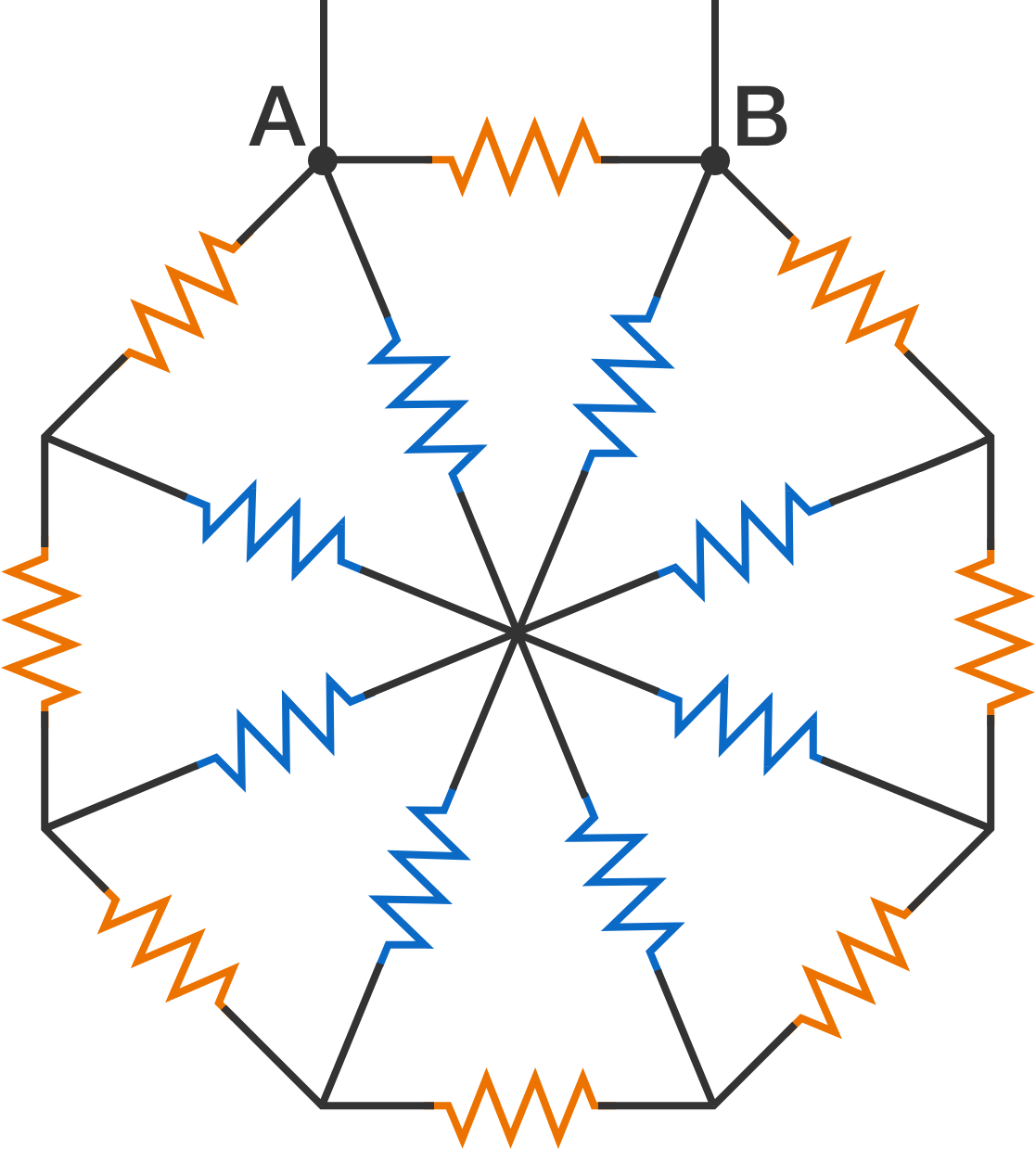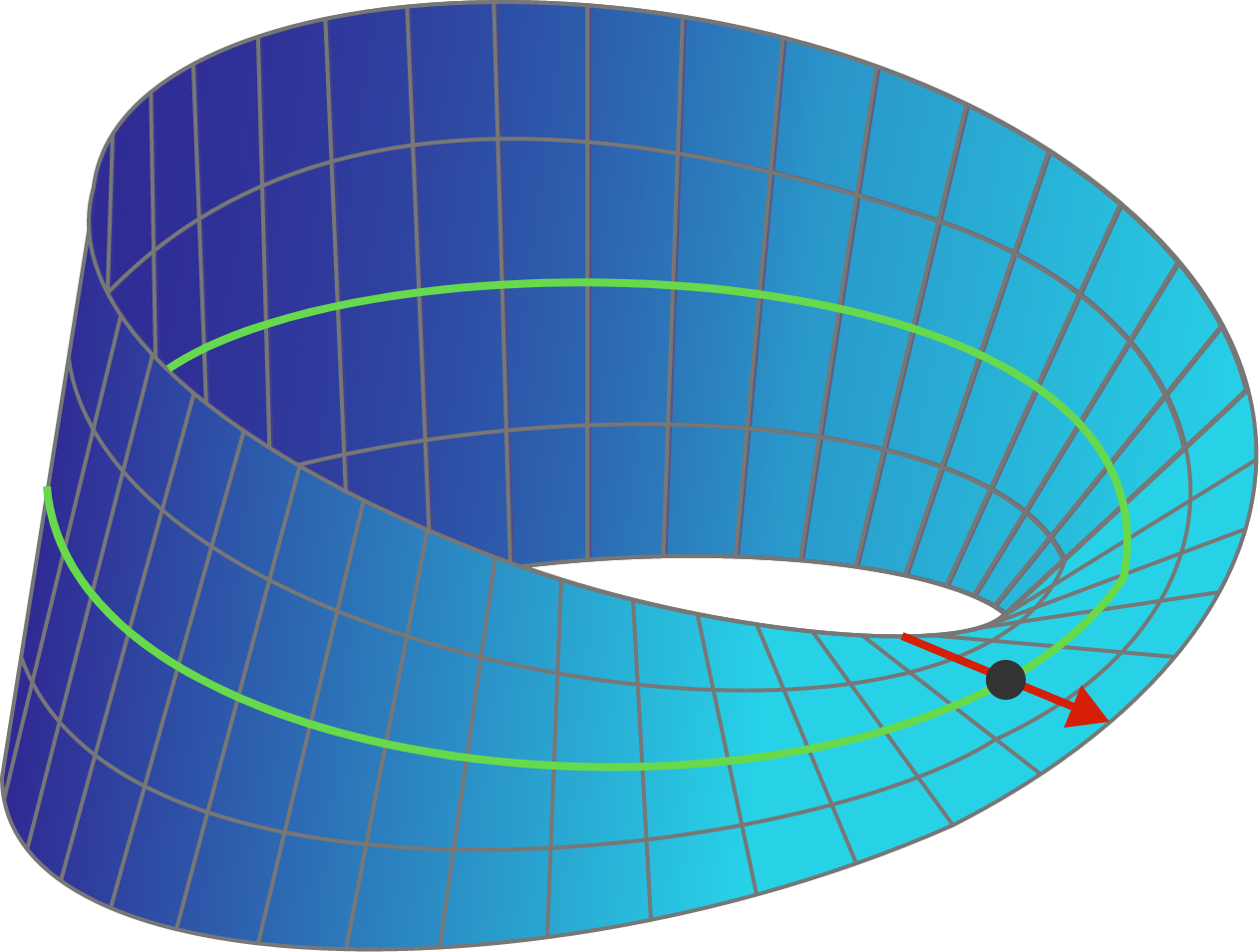# 2017-04-03 Advanced

Does there exist a continuous function $f : \mathbb{R}^2 \to \mathbb{R}$ that is also injective?

An injective function is one-to-one, meaning that no element of the range is the image of more than one element of the domain.8 resistors (orange color) are connected to form a regular octagon. 8 more resistors (blue color) connect the vertices of the octagon to its center. All the 16 resistors are of resistance $\SI{420}{\ohm}$.

If the connecting wires have negligible resistance, calculate the equivalent resistance (in ohms, rounded to the nearest integer) between the terminals $A$ and $B$.

Find the smallest positive integer $n$ for which

$n^{50} + (n+1)^{50} \gt (n+2)^{50}.$

Inspiration

Consider a Mobius strip whose width (the red line) is extended indefinitely in both directions throughout 3D space. Note that the Mobius surface will intersect itself.Consider all the regions that this surface would divide 3D space into. How many (disconnected) regions are there? And how many of them have an infinite volume?

Enter your answer as $a . b$, where $a$ is the number of disconnected regions, and $b$ is the number of regions which have infinite volume. For example, if you believe there are 4 regions, of which 3 have infinite volume, enter your answer as $4.3$.

Formally, the Mobius surface as shown in the graphic above is defined as a ruled surface, with a circle as the directrix curve (green), through each point on which (big black point) passes a line (red) perpendicular to the tangent of the circle at that point. As one progresses around the directrix circle, the angle that line makes with the plane of the directrix circle varies by exactly half of the angle around the directrix circle, so that after it has gone around once, the arrow points in the opposite direction, and going around one more time brings the arrow back to the original orientation. We are then extending the red line indefinitely in both directions.

On a large $999 \times 999$ board, each cell is colored either white or black.

Define a coordinated triple of cells $(C_1, C_2, C_3 )$ as the formation such that

• $C_1$ and $C_2$ are in the same row;
• $C_2$ and $C_3$ are in the same column;
• $C_1$ and $C_3$ are white;
• $C_2$ is black.

What is the maximum number of coordinated triples? Enter your answer as the last three digits of that maximum.

×

Problem Loading...

Note Loading...

Set Loading...